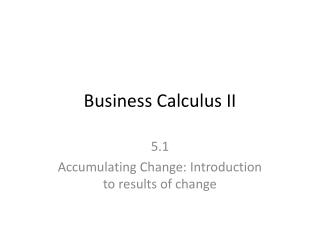DownloadDownload PresentationDownload Presentation- - - - - - - - - - - - - - - - - - - - - - - - - - - E N D - - - - - - - - - - - - - - - - - - - - - - - - - - -
##### Presentation Transcript

1. Business Calculus II 5.1 Accumulating Change: Introduction to results of change

2. Accumulated Change • If the rate-of-change function f’ of a quantity is continuous over an interval a<x<b, the accumulated change in the quantity between input values of a and b is the area of the region between the graph and horizontal axis, provided the graph does not crosses the horizontal axis between a and b. • If the rate of change is negative, then the accumulated change will be negative. • Example: • Positive- distance travel • Negative-water draining from the pool

3. 5.1 – Accumulated Distance (PAGE 319)

4. Accumulated Change involving Increase and decrease • Calculate positive region (A) • Calculate negative region (B) • Then combine the two for overall change

5. Maximum Rate of Change (ROC) Function Behavior Minimum Positive Slope Negative Slope Positive Slope Zero Zero

6. Rate of Change (ROC)Function Behavior Inflection Point Concave Down Decreasing Concave Up Increasing

7. Problems 2, 6, 7, 12 (pages 324-328)

8. Business Calculus II 5.2 Limits of Sums and the Definite Integral

9. Approximating Accumulated Change • Not always graphs are linear! • Left Rectangle approximation • Right Rectangle approximation • Midpoint Rectangle approximation

10. Left Rectangle approximation

11. Sigma Notation • When xm, xm+1, …, xn are input values for a function f and m and n are integers when m<n, the sum f(xm)+f(xm+1)+….f(xn)can be written using the greek capital letter sigma () as

12. Right Rectangle approximation

13. Mid-Point Rectangle approximation

14. Area Beneath a Curve • Area as a Limit of Sums • Let f be a continuous nonnegative function from a to b. The area of the region R between the graph of f and x-axis from a to b is given by the limit Where xi is the midpoint of the ith subinterval of length x= (b-a)/n between a and b.

15. Page 334- Quick Example • Calculator Notation for midpoint approximation:Sum(seq(function * x, x, Start, End, Increment) • Start: a + ½ x • End: b - ½ x • Increment: x

16. Left rectangle • Calculator Notation :Sum(seq(function * x, x, Start, End, Increment) • Start: a • End: b - x • Increment: x

17. Right Rectangle • Calculator Notation:Sum(seq(function * x, x, Start, End, Increment) • Start: a + x • End: b • Increment: x

18. Related Accumulated Change to signed area • Net Change in Quantity • Calculate each region and then combine the area.

19. Definite Integral • Let f be a continuous function defined on interval from a to b. the accumulated change (or definite Integral) of f from a to b is Where xi is the midpoint of the ith subinterval of length x= (b-a)/n between a and b.

20. Problems 2, 8 (pages 338-342)

21. Business Calculus II 5.3 Accumulation Functions

22. Accumulation Function • The accumulation function of a function f, denoted by gives the accumulation of the signed area between the horizontal axis and the graph of f from a to x. The constant a is the input value at which the accumulation is zero, the constant a is called the initial input value.

23. 2. Velocity (page 350)

24. 4. Rainfall (page 351)

25. Using Concavity to refine the sketch of an accumulation Function (Page 348) Faster Slower Increase decrease Increase decrease Slower Faster

26. Graphing Accumulation Function using F’ When F’ Graph has x-intercept, then you have Max/Min/inflection point in accumulation graph How to identify the critical value(s): MAX in Accumulation graph: When F’ graph changes from Positive to negative MIN in Accumulation graph: When f’ graph changes from negative to positive Inflection point in accumulation graph: When F’ touches the x-axis Or You have MAX/MIN in F’ graph

27. Graphing Accumulation Function using F’ Max: Positive to negative Positive F’ x-intercept, MAX – in Accumulation graph Negative F’

28. Graphing Accumulation Function using F’ Min: negative to Positive Positive F’ x-intercept, MIN – in Accumulation graph   Negative F’

29. Graphing Accumulation Function using F’ Inflection Point: F’ Touches the x-axis x-intercept, MIN – in Accumulation graph

30. Graphing Accumulation Function using F’ Inflection Point: inflection point in F’, also appears as inflection point in accumulation graph Inflection Points in F’

31. WHAT WE HAVE COMBINE INF INF MAX MIN INF INF INF

32. Positive area Start at zero

33. 10-Sketch

34. 12-sketch

35. 14-sketch

36. Business Calculus II 5.4 Fundamental Theorem

37. Fundamental Theorem of Calculus (Part I) For any continuous function f with input x, the derivative of in term use of x: FTC Part 2 appears in Section 5.6.

38. Anti-derivativeReversal of the derivative process Let f be a function of x . A function F is called an anti-derivative of f if That is, F is an anti-derivative of f if the derivative of F is f.

39. General and Specific Anti-derivative • For f, a function of x and C, an arbitrary constant, is a general anti-derivative of f When the constant C is known, F(x) + C is a specific anti-derivative.

40. Simple Power Rule for Anti-Derivative

41. More Examples:

42. Constant Multiplier Rule for Anti-Derivative

43. Sum Rule and Difference Rule for Anti-Derivative

44. Example:

45. Connection between Derivative and Integrals • For a continuous differentiable function fwith input variable x,

46. Example:

47. Problem: 2,12,14,16,20,22,24,37

48. Business Calculus II 5.5 Anti-derivative formulas for Exponential, LN

49. 1/x(or x-1) Rule for Anti-derivative ex Rule for Anti-derivative ekx Rule for Anti-derivative

50. Exponential Rule for Anti-derivative Natural Log Rule for Anti-derivative Please note we are skipping Sine and Cosine Models